Search

Conics: Circles: Further Examples (page 3 of 3)

Sections: Introduction & Drawing, Working with equations, Further examples• Find an equation for the circle centered at (1, 5)
and tangent to the line
3x + 4y = 8.

For one line (or curve) to be "tangent" to another means that the lines just touch; they don't cross. In the context of circles, the tangent is perpendicular to the radius line at that point on the circle. This means that we need to find the line perpendicular to 3x + 4y = 8 and passing through the point (1, 5), because the radius will lie on that line. Also, the intersection of the radius line and the given line will be a point on the circle, from which we can find the length of the radius.

First, I find the slope of the given line:

Advertisement

3x + 4y = 8
4y = 3x + 8

y = (3/4)x + 2

From the slope-intercept form of the line, I can see that the slope of the given line is 3/4, so my radius line has slope m = 4/3. Plugging this and the center point into the point-slope equation of a straight line, I get:

y  (5) = (4/3)(x  1)
y + 5 = (4/3)(x  1)

y = (4/3)x  (19/3)

The intersection of the radius line and the tangent line is a point on the circle, so I'll solve the system of equations represented by these two lines:

(3/4)x + 2 = (4/3)x  (19/3)
9x + 24 = 16x  76

24+76 = 16x + 9x

100 = 25x

4 = x

Then y = 1, and the point (4, 1) is on the circle. The Distance Formula gives me the length of the radius: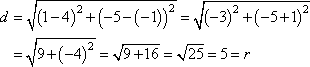So r = 5, and my equation is:

(x  1)2 + (y + 5)2 = 25

The "interior" of a circle is all the points inside the circle's line. Algebraically, the distance of an interior point from the center is less than the value of the radius. The "exterior" is all points outside the circle's line, having distances from the center greater than the radius.

• Is the point (2, 0) in the interior or exterior of the circle
with equation
(x  3)2 + (y + 5)2 = 49?
• The center of the circle is (h, k) = (3, 5) and the radius is r = 7. To determine on which "side" of the circle this point lies, I need to find its distance from the center.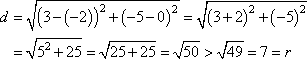Since this distance is more than the radius, then this point is in the exterior.

To check your graphs, or to graph an equation to verify that it fits the requirements of an exercise, solve the circle equation for the two half-circle equations. For instance, (x  3)2 + (y + 2)2 = 25 solves as: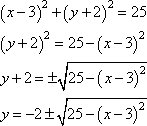The "plus the square root of" part is the top half of the circle; the "minus the square root of" part is the bottom half. Plug these two halves into your calculator as follows: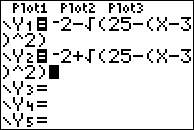...and graph: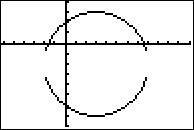The standard calculator set-up, with the tickmarks on the x-axis being wider apart than on the y-axis, will make the graph look squashed; the above was done using the "Zoom-Square" setting. Even squashed, though, your graph should suffice to confirm that the circle is centered at or near (3, 2), with a radius at or near 5. Copyright © Elizabeth Stapel 2010-2011 All Rights Reserved

Make sure you memorize the center-radius form of the equation, and practice how to complete the square, how to do quick but nicely round circle graphs, and how to use your graphing calculator to check your answers. Other than involving some messy computations, circles aren't too bad  as conics go.

<< Previous  Top  |  1 | 2 | 3  |  Return to Index

 Cite this article as: Stapel, Elizabeth. "Conics: Circles: Further Examples." Purplemath. Available from     https://www.purplemath.com/modules/circle3.htm. Accessed [Date] [Month] 2016

Study Skills Survey

Tutoring from Purplemath
Find a local math tutor

 Copyright © 2021  Elizabeth Stapel   |   About   |   Terms of Use   |   Linking   |   Site Licensing Contact Us